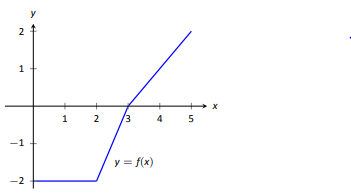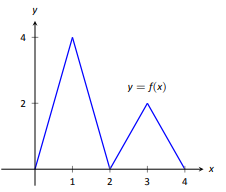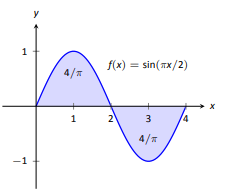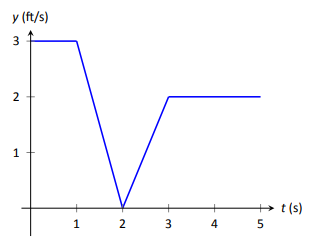$$\newcommand{\id}{\mathrm{id}}$$ $$\newcommand{\Span}{\mathrm{span}}$$ $$\newcommand{\kernel}{\mathrm{null}\,}$$ $$\newcommand{\range}{\mathrm{range}\,}$$ $$\newcommand{\RealPart}{\mathrm{Re}}$$ $$\newcommand{\ImaginaryPart}{\mathrm{Im}}$$ $$\newcommand{\Argument}{\mathrm{Arg}}$$ $$\newcommand{\norm}{\| #1 \|}$$ $$\newcommand{\inner}{\langle #1, #2 \rangle}$$ $$\newcommand{\Span}{\mathrm{span}}$$

# 4.2E: Exercises

$$\newcommand{\vecs}{\overset { \rightharpoonup} {\mathbf{#1}} }$$ $$\newcommand{\vecd}{\overset{-\!-\!\rightharpoonup}{\vphantom{a}\smash {#1}}}$$$$\newcommand{\id}{\mathrm{id}}$$ $$\newcommand{\Span}{\mathrm{span}}$$ $$\newcommand{\kernel}{\mathrm{null}\,}$$ $$\newcommand{\range}{\mathrm{range}\,}$$ $$\newcommand{\RealPart}{\mathrm{Re}}$$ $$\newcommand{\ImaginaryPart}{\mathrm{Im}}$$ $$\newcommand{\Argument}{\mathrm{Arg}}$$ $$\newcommand{\norm}{\| #1 \|}$$ $$\newcommand{\inner}{\langle #1, #2 \rangle}$$ $$\newcommand{\Span}{\mathrm{span}}$$ $$\newcommand{\id}{\mathrm{id}}$$ $$\newcommand{\Span}{\mathrm{span}}$$ $$\newcommand{\kernel}{\mathrm{null}\,}$$ $$\newcommand{\range}{\mathrm{range}\,}$$ $$\newcommand{\RealPart}{\mathrm{Re}}$$ $$\newcommand{\ImaginaryPart}{\mathrm{Im}}$$ $$\newcommand{\Argument}{\mathrm{Arg}}$$ $$\newcommand{\norm}{\| #1 \|}$$ $$\newcommand{\inner}{\langle #1, #2 \rangle}$$ $$\newcommand{\Span}{\mathrm{span}}$$

## Exercise $$\PageIndex{1}$$

1. What is "total signed area"?

2. What is "displacement"?

3. What is $$\int_3^3 \sin x\,dx$$

4. Give a single definite integral that has the same value as $$\int_0^1 (2x+3)\,dx +\int_1^2 (2x+3)\,dx$$.

Under Construction

## Exercise $$\PageIndex{2}$$

A graph of a function $$f(x)$$ is given. Using the geometry of the graph, evaluate the definite integrals.

1.(a) $$\int_0^1 (-2x+4)\,dx$$
(b) $$\int_0^2 (-2x+4)\,dx$$
(c) $$\int_0^3 (-2x+4)\,dx$$
(d) $$\int_1^3 (-2x+4)\,dx$$
(e) $$\int_2^4 (-2x+4)\,dx$$
(f) $$\int_0^1 (-6x+12)\,dx$$

2.(a) $$\int_0^2 f(x)\,dx$$
(b) $$\int_0^3 f(x)\,dx$$
(c) $$\int_0^5 f(x)\,dx$$
(d) $$\int_2^5 f(x)\,dx$$
(e) $$\int_5^3 f(x)\,dx$$
(f) $$\int_0^3 f(x)\,dx$$

3.(a) $$\int_0^2 f(x)\,dx$$
(b) $$\int_2^4 f(x)\,dx$$
(c) $$\int_2^4 2f(x)\,dx$$
(d) $$\int_0^1 4x\,dx$$
(e) $$\int_2^3 (2x-4)\,dx$$
(f) $$\int_2^3 (4x-8)\,dx$$

4.(a) $$\int_0^1 (x-1)\,dx$$
(b) $$\int_0^2 (x-1)\,dx$$
(c) $$\int_0^3 (x-1)\,dx$$
(d) $$\int_2^3 (x-1)\,dx$$
(e) $$\int_1^4 (x-1)\,dx$$
(f) $$\int_1^4 \left ((x-1)+1\right )\,dx$$

5.(a) $$\int_0^2 f(x)\,dx$$
(b) $$\int_2^4 f(x)\,dx$$
(c) $$\int_0^4 f(x)\,dx$$
(d) $$\int_0^4 5f(x)\,dx$$

Under Construction

## Exercise $$\PageIndex{3}$$

A graph of a function $$f(x)$$ is given; the numbers inside the shaded regions give the area of that region. Evaluate the definite integrals using this area information.

1.(a) $$\int_0^1 f(x)\,dx$$
(b) $$\int_0^2 f(x)\,dx$$
(c) $$\int_0^3 f(x)\,dx$$
(d) $$\int_1^2 -3f(x)\,dx$$

2.(a) $$\int_0^2 f(x)\, dx$$
(b) $$\int_2^4 f(x)\, dx$$
(c) $$\int_0^4 f(x)\, dx$$
(d) $$\int_0^1 f(x)\, dx$$

3.(a) $$\int_{-2}^{-1}f(x)\,dx$$
(b) $$\int_{1}^{2}f(x)\,dx$$
(c) $$\int_{-1}^{1}f(x)\,dx$$
(d) $$\int_{0}^{1}f(x)\,dx$$

4.(a) $$\int_0^2 5x^2\,dx$$
(b) $$\int_0^2 (x^2+1)\,dx$$
(c) $$\int_1^3 (x-1)^2\,dx$$
(d) $$\int_2^4 \left ( (x-2)+5\right )\,dx$$

Under Construction

## Exercise $$\PageIndex{4}$$

A graph of the velocity function of an object moving in a straight line is given. Answer the questions based on that graph.

1.(a) What is the object's maximum velocity?
(b) What is the object's maximum displacement?
(c) What is the object's total displacement on [0,3]?

2.(a) What is the object's maximum velocity?
(b) What is the object's maximum displacement?
(c) What is the object's total displacement on [0,5]?

Under Construction

## Exercise $$\PageIndex{5}$$

An object is thrown straight up with a velocity, in ft/s, given by $$v(t) = -32t+64$$, where $$t$$ is in seconds, from a height of 48 feet.
(a) What is the object's maximum velocity?
(b) What is the object's maximum displacement?
(c) When does the maximum displacement occur?
(d) When will the object reach a height of 0? (Hint: find when the displacement is -48ft.)

Under Construction

## Exercise $$\PageIndex{6}$$

An object is thrown straight up with a velocity, in ft/s, given by $$v(t)=-32t+96$$, where $$t$$ is seconds, from a height of 64 feet.
(a) What is the object's initial velocity?
(b) What is the object's displacement 0?
(c) How long does it take for the object to return to its initial height?
(d) When will the object reach a height of 210ft?

Under Construction

## Exercise $$\PageIndex{7}$$

Use these values to evaluate the given definite integrals.

• $$\int_0^2 f(x) \,dx=5$$,
• $$\int_0^3 f(x) \,dx=7$$,
• $$\int_0^2 g(x) \,dx=-3$$, and
• $$\int_2^3 g(x) \,dx=5$$.

1. $$\int_0^2 \left ( f(x)+g(x)\right )\,dx$$

2. $$\int_0^3 \left ( f(x)-g(x)\right )\,dx$$

3. $$\int_2^3 \left ( 3f(x)+2g(x)\right )\,dx$$

4. Find values for $$a$$ and $$b$$ such that
$$\int_0^3 \left ( af(x)+bg(x)\right )\,dx=0$$

Under Construction

## Exercise $$\PageIndex{8}$$

Use these values to evaluate the given definite integrals.

• $$\int_0^3 s(t)\,dt =10$$,
• $$\int_3^5 s(t)\,dt =8$$,
• $$\int_3^5 r(t)\,dt =-1$$, and
• $$\int_0^5 r(t)\,dt =11$$.

1. $$\int_0^3 \left ( s(t)+r(t)\right )\,dt$$

2. $$\int_5^0 \left ( s(t)-r(t)\right )\,dt$$

3. $$\int_3^3 \left ( \pi s(t)-7r(t)\right )\,dt$$

4. Find values for a and b such that
$$\int_0^5 \left ( ar(t)+bs(t)\right )\,dt=0$$

Under Construction

## Exercise $$\PageIndex{9}$$

Evaluate the given indefinite integral:

1. $$\int (x^3-2x^2+7x-9)\,dx$$

2. $$\int (\sin x -\cos x +\sec^2 x)\,dx$$

3. $$\int (\sqrt{t}+\frac{1}{t^2}+2^t)\,dt$$

4. $$\int \left ( \frac{1}{x} -\csc x \cot x \right )\,dx$$